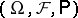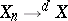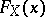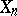# Convergence in distribution

(diff) ← Older revision | Latest revision (diff) | Newer revision → (diff)

Convergence of a sequence of random variablesdefined on a certain probability space, to a random variable, defined in the following way:if(*)

for any bounded continuous function. This form of convergence is so called because condition (*) is equivalent to the convergence of the distribution functionsto the distribution functionat every pointat whichis continuous.

This is special terminology for real-valued random variables for what is generally known as weak convergence of probability measures (same definition as in (*), but with,taking values in possibly more general spaces).